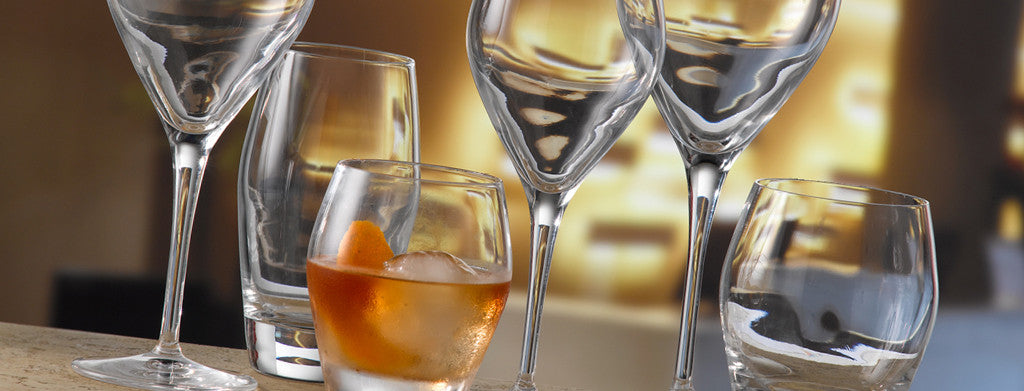Shopping Cart

### Refine

View all

#### Price# Glassware£2.62 each (ex VAT)£3.45 each (ex VAT)£3.88 each (ex VAT)£3.72 each (ex VAT)£3.72 each (ex VAT)£2.92 each (ex VAT)£3.53 each (ex VAT)£3.72 each (ex VAT)£3.72 each (ex VAT)£5.36 each (ex VAT)£6.78 each (ex VAT)£5.74 each (ex VAT)£5.74 each (ex VAT)£5.74 each (ex VAT)£4.91 each (ex VAT)£4.40 each (ex VAT)£5.53 each (ex VAT)£5.74 each (ex VAT)£3.25 each (ex VAT)£3.35 each (ex VAT)£2.97 each (ex VAT)£3.63 each (ex VAT)£3.53 each (ex VAT)£3.02 each (ex VAT)£3.63 each (ex VAT)£2.62 each (ex VAT)£3.76 each (ex VAT)£3.35 each (ex VAT)£3.02 each (ex VAT)